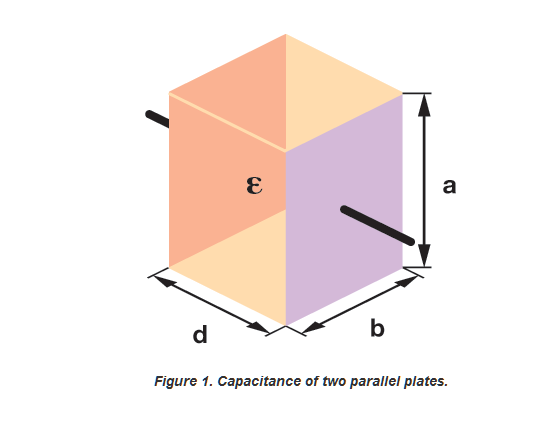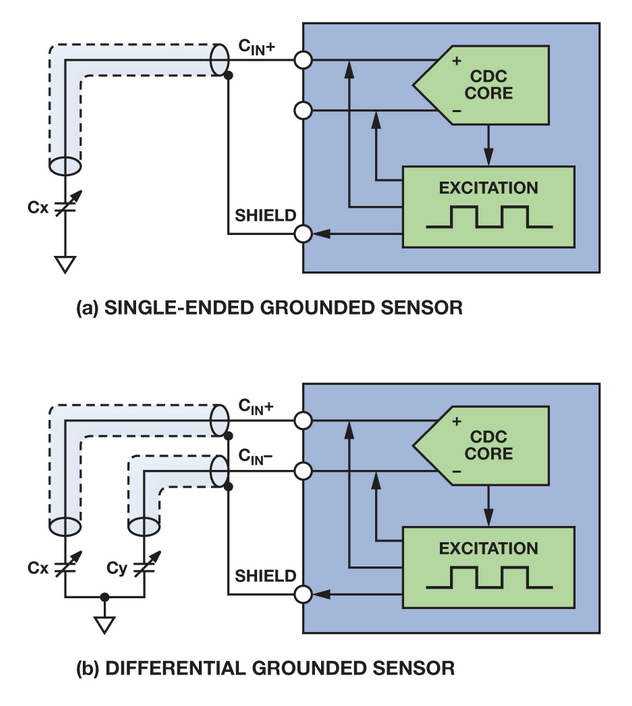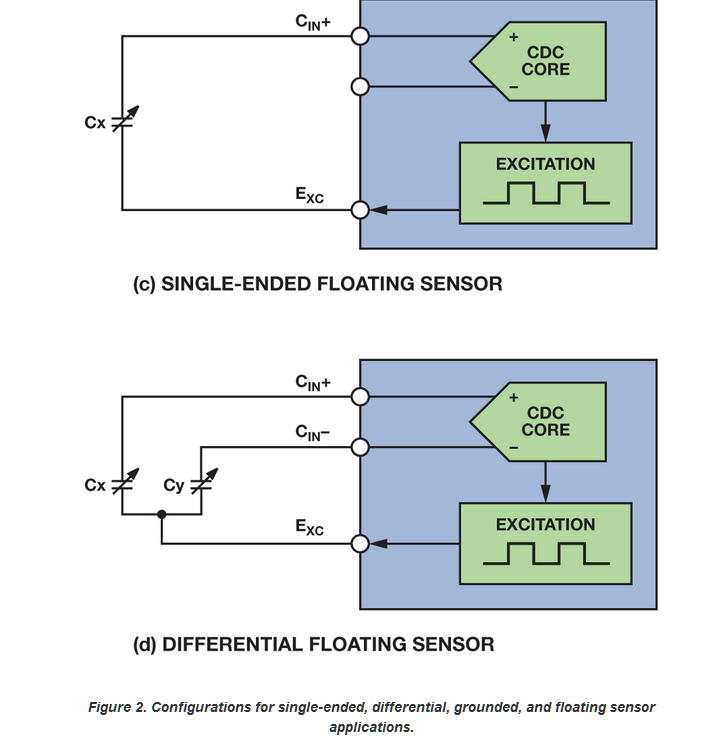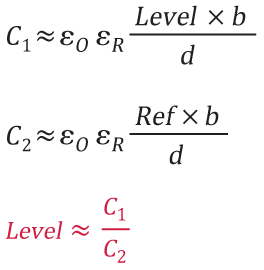## Liquid Level Sensing Using Capacitive-to-Digital Converters

### Introduction

Procedures such as infusions and transfusions require exact amounts of liquid to be monitored, so they need an accurate, easy-to-implement method for sensing liquid level. This article describes the 24-bit capacitive-to-digital converters and level-sensing techniques that enable high-performance capacitive sensing of liquid levels.

### Capacitance Measurement Basics

Capacitance is the ability of a body to store electrical charge. The capacitance, C, is given by

where Q is the charge on the capacitor and V is the voltage across the capacitor.

In the capacitor shown in Figure 1, two parallel metal plates with area A are separated by distance d. The capacitance, C, is

where

• C is the capacitance in Farads
• A is the area of overlap of the two plates = a × b
• d is the distance between the two plates
• εR is the relative static permittivity
• εO is the permittivity of free space (εO ≈ 8.854 × 10−12 F m−1)### Capacitance-to-Digital Converter (CDC)

The single-channel AD7745 and two-channel AD7746 high-resolution, Σ-Δ capacitance-to-digital converters measure capacitances connected directly to their inputs. Featuring inherently high resolution (21-bit effective resolution and no missing codes at 24 bits), high linearity (±0.01%), and high accuracy (±4 fF factory calibrated), they are ideal for sensing levels, position, pressure, and other physical parameters.

Functionally complete, they integrate a multiplexer, an excitation source, switched-capacitor DACs for the capacitive inputs, a temperature sensor, a voltage reference, a clock generator, control and calibration logic, an I2C-compatible serial interface, and a high-precision converter core, which includes a second-order Σ-Δ charge-balancing modulator and a third-order digital filter. The converter works as a CDC for capacitive inputs and as an ADC for voltage inputs.

The measured capacitance, Cx, is connected between the excitation source and the Σ-Δ modulator input. A square-wave excitation signal is applied to Cx during the conversion. The modulator continuously samples the charge going through the Cx and converts it to a stream of 0s and 1s. The digital filter processes the modulator output to determine the capacitance, which is represented by the density of 1s. The filter output is scaled by calibration coefficients. The external host can then read the final result via the serial interface.

The four configurations shown in Figure 2 demonstrate how the CDC senses capacitance in single-ended, differential, grounded, and floating sensor applications.### Capacitive Level-Sensing Techniques

A simple technique for monitoring liquid levels is to immerse a parallel-plate capacitor in the liquid, as shown in Figure 3. As the liquid level changes, the amount of dielectric material between the plates changes, which causes the capacitance to change as well. A second pair of capacitive sensors (shown as C2) is used as a reference.Figure 4. Capacitive level-sensing system.

Post time: Jul-25-2020